• 2021-11-04 14:47:48

# 信号采样与sinc插值恢复[MATLAB]

根据采样定理，若想无失真地从采样信号重建信号，需要满足：

• 信号为频带有限信号。

• f s ≥ 2 f m f_s \ge 2f_m

令插值因子为sinc函数
h ( m ) = s i n c ( m ) = s i n ( π m ) π m h(m) = sinc(m) = \frac{sin(\pi m)}{\pi m}
则插值信号为
g ( m ) = ∑ i g d ( i ) s i n c ( m − i ) g(m) = \sum_i g_d(i)sinc(m-i)
下面通过MATLAB进行仿真，代码如下：

%参数设定
T = 2;
f1 = 5;
f2 = 10;
fs = 40;
%可得参数
N = T * fs;
t = linspace(-T/2, T/2, 100*N);
f_ori = 0.5 * cos(2 * pi * f1 * t) - sin(2 * pi * f2 * t) + 1;
figure(1);
plot(t, f_ori);xlim([-T/10, T/10]);title('原始信号');
%采样
t2 = linspace(-T/2, T/2, N);
f_sam = 0.5 * cos(2 * pi * f1 * t2) - sin(2 * pi * f2 * t2) + 1;
figure(2);
stem(t2, f_sam);xlim([-T/10, T/10]);title('采样信号');
%恢复
y = [];
for i = 1 : length(t)
x = t(i);
h = sinc((x - t2).*fs);
g = dot(f_sam, h);
y = [y,g];
end
figure(3);
plot(t, y);xlim([-T/10, T/10]);title('恢复信号');


结果如下：matlab 数字信号处理
更多相关内容
• 很好地插值函数，可以直接调用，简单易懂，注释清晰。
• sinc插值程序_sinc函数插值_sinc_sinc插值_sinc插值_sinc插值实例_源码.zip
• sinc插值和最近领域插值完成距离弯曲校正的完整程序和几篇弯曲校正文章
• 很好地插值函数，可以直接调用，简单易懂，注释清晰。matlab
• 1.时域信号 ...2.时域5倍sinc插值后 实部： 相位： 3.将时域信号转换为频域，在频域补零后ifft实现5倍插值 实部： 相位： 结论： 通过matlab仿真验证了sinc时域卷积和频域补零实现插值的等效性。 ...

1.时域信号
a=exp(1jpi(-4:0.08:4).^2)
实部：2.时域5倍sinc插值后
实部：相位：3.将时域信号转换为频域，在频域补零后ifft实现5倍插值
实部：相位：结论：
通过matlab仿真验证了sinc时域卷积和频域补零实现插值的等效性。

展开全文matlab
• 实验一 基于 MATLAB 的白噪声信号 u(n)sinc 函数chirp 信号产生实验 一 实验目的 1. 学会使用 MATLAB 2. 通过实验了解 MATLAB 如何产生各种常用信号 3. 掌握 MATLAB 的编程方法 二 实验内容 1. 用 MATLAB 编程产生一...
• 合成孔径雷达成像RD算法中用sinc插值校正距离弯曲的程序 经编写并调试后上传，可以正常应用。CSDN把下载所需积分调的太高（50），我来调少些。
• sinc 函数内插 我们的信号 x(t) 是个实信号，带宽有限，能量有限。x[n] =x(nΔ)和 x’[n] =x(nΔ’)是对这个信号的两种采样，并且都满足采样定理的要求，也就是说信息并没有丢失。两次采样的采样率满足如下...

有些时候，为了后续处理更方便，我们需要对采集到的数据点进行内插处理，也就是所谓的增采样。本文就来讨论一下常用的几种内插算法。

## sinc 函数内插

我们的信号 x(t) 是个实信号，带宽有限，能量有限。x[n] =x(nΔ)和 x’[n]  =x(nΔ’)是对这个信号的两种采样，并且都满足采样定理的要求，也就是说信息并没有丢失。两次采样的采样率满足如下关系。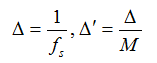也就是说第二种采样的采样率是第一种采样的采样率的倍。如果我们有了x’[n]，那么很容易获得x[n]：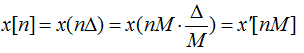但是反过来就不是那么容易了。下面就来推导如何在已知x[n]的情况下，计算出x’[n]。

对于连续信号x(t)，有如下关系。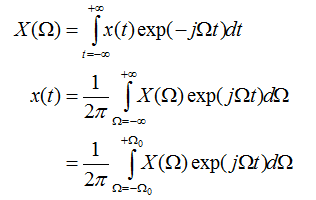对于离散信号x[n]，有如下关系。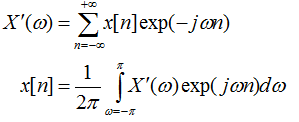这里的ω与Ω的关系如下：ω=ΩΔ，那X’(ω)与X(Ω)有什么关系呢？我们先假设X’(ω)=X(Ω)，那么有：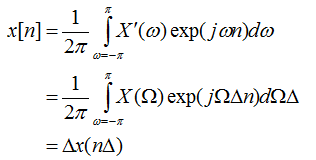这说明，X’(ω)=X(Ω)/Δ。利用这个关系式，可以得到：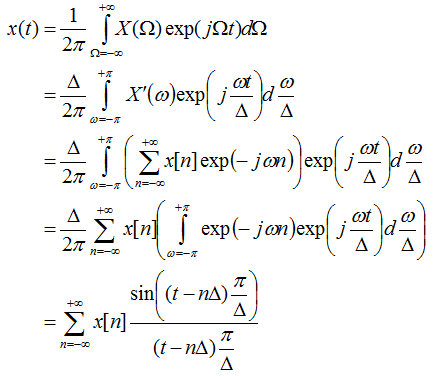至此，利用sinc函数内插的公式就出来了：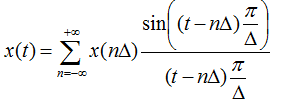当Δ=1时，上面式子还可以简化为：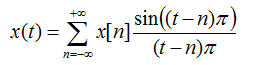下面是个测试例子，scilab 语言的。

function y = sinc_interp(x, n)
s = size(x);
if s(1) == 1 then
nx = s(2);
ny =  (nx) * n;
s(2) = ny;
else
nx = s(1);
ny =  (nx) * n;
s(1) = ny;
end
y = zeros(s(1), s(2));

for(i = 1 : ny)
t = (i - 1) / n + 1;
a = ceil( t - 5 );
b =  floor(t + 5);
if (a < 1) then a = 1;  end
if (b > nx) then b = nx;  end
if t == int(t) then
y(i)  = x(t);
else
z = 0;
for j =a : b
z = z + x(j) * sin((t-j)*%pi) / ((t-j)*%pi);
end
y(i) = z;
end
end
endfunction

用这个函数随便测试了一个随机生成的数列的内插结果。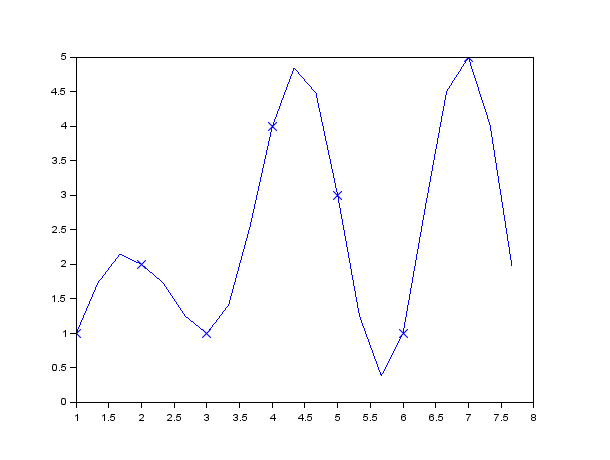再将这个结果与 fft 内插的结果比较一下，可以看到效果类似，之所以不完全相同，是因为这个代码中只用到了临近的5个数据点，而且到了边界处就直接截断处理了。而fft内插是将数据周期延拓后计算的结果。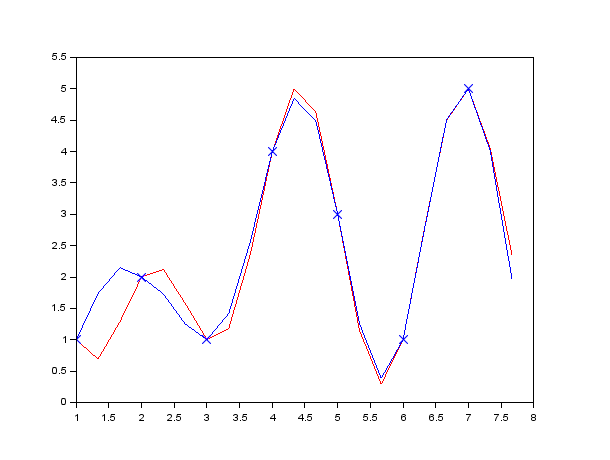展开全文• 在matlab中对二维sinc函数进行了模拟，画出了图像。横纵坐标为-5至5.
• 在matlab中对二维sinc函数进行了模拟，画出了图像。谢谢大家的支持！
• 前言自从接触到数字信号，特别是采样定理之后，我就一直非常好奇一个问题：两个相邻的采样点之间，要以什么样的形式，怎么样连接起来？...直到接触到了sinc函数(又称为抽样函数，Sa函数，辛格函数)...

前言

自从接触到数字信号，特别是采样定理之后，我就一直非常好奇一个问题：两个相邻的采样点之间，要以什么样的形式，怎么样连接起来？

因为正弦波才是频率最单一的波形，如果两个采样点直接用直线连接(变成了三角波)，那么又能产生更高频率的信号了，显然跟采样定理是相悖的。

所以我就想，是不是有一种插值的方法，在两个采样点之间的插值形成的是一条正线曲线？

直到接触到了sinc函数(又称为抽样函数，Sa函数，辛格函数)，才豁然开朗。

小小的一个从采样点还原波形的过程，就蕴含了这么多知识。

将模拟信号转换为数字信号的过程，在信号处理领域叫做采样(Sampling)。我们可以形象地把这个过程理解为使用一连串宽度非常窄的脉冲和输入信号相乘。而得到的结果则是一连串时间上不连续的脉冲。

而当我们需要从数字设备里将音频播放出来，我们就需要进行一个相反的过程。数据被传送到声卡上，经过DA芯片进行数模转换之后，输出可以连接到扬声器上的模拟电信号。这时候，就需要一个办法，来将离散的点变得连续起来了。

从时域到频域

在对信号进行处理的过程中，我们经常使用傅立叶变换。傅立叶变换将信号从时域转到频域，便于分析和处理。

当采样脉冲的宽度越来越窄，采样后的信号具有的频谱宽度会越来越宽。在理论分析时，我们可以假设脉冲的宽度趋于0，也就是δ函数。这时候信号的频谱在频域上无限重复延展。我们在还原信号的时候，只需要在频谱上做一个低通滤波，把那些延展出来的频率过滤掉，得到的就是原始的信号啦！

而根据傅立叶变换的性质，在频域上乘积，等价于在时域上的卷积。而低通滤波器，可以近似看为一个矩形函数。矩形函数的傅立叶变换(或者逆变换)，则是Sinc函数。所以，低通滤波的操作，又相当于把采样点和Sinc函数进行了卷积。采样点和采样点之间的曲线，也就自然而然地形成了。

直观地感受卷积的过程

写了段代码来展示卷积操作的这个过程。

简直太漂亮了好吗！！！入迷了！！！由于卷积跟信号的顺序没有关系，互换一下两个函数，虽然看起来不那么直观，但是也是很炫酷的一个过程，嘻嘻再来看看使用卷积生成其他函数实际上，DA的的输出电路里，我们会使用一个模拟低通滤波器来完成这项把离散的采样点变成连续的输出波形的工作。

了解更多音频知识：

展开全文• ## 二维SINC函数

千次阅读 2019-11-04 19:00:16
画二维SINC函数 MATLAB clear;clc x=linspace(-10,10,200); y=linspace(-10,10,200); for i=1:length(x) for j=1:length(y) x1=x(i)*pi; y1=y(j)*pi; x2=sin(x1)/x1; y2=sin(...
• 为了内容的完整性，这里简单的介绍了sinc函数，这个函数的更多应用实在信号处理中，其他方便不清楚，因此，先基本了解，之后关于采样函数的重构等知识在相关学科中再了解吧。这是一个最基本的例子，画出来sinc函数的...
• 我有一个3D数据集和一个2D数据集,它是第一个卷的切片....下面的第一条评论已经指出我在matlab中执行线性插值的现有函数,但我也想知道如何自己这样做,所以我可以使用sinc插值(和其他).我可以对坐标进行舍入并获...
• 这是一个sinc加窗滤波器，截止频率为0.25，实现对输入的信号进行低通滤波的效果matlab
• 当已知数据点位于整数格点上时，插值函数h(x)应该有：h(0)=1;h(n)=0; n是非零整数。 在频率域，以步长1采样的图像中如果含有高于1/2的频率分量将会产生混叠。空间域内的卷积对应在频率域内的相乘运算，可以...
• ## sinc函数

千次阅读 2015-10-07 20:12:00
在频域它的形状象一个矩形函数，在时域它的形状象一个Sinc函数。由于理想的 Sinc 滤波器（人们熟知的矩形滤波器）有无限的延迟，所以现实世界中的滤波器只能是它的一个近似，但是它仍然在概念演示或者验证中得到了...
• %插值和重采样函数，其中： % I = 插值率% D = 重采样位置，要移动的样本数% x(n) = 输入波形% y(n*(1/I) + D) = 输出波形% --包括具有指数衰减函数的选项平滑以减少吉布斯振铃matlab
• 使用 SINC 内核对原始输入时间序列进行卷积以获得重采样时间序列的稳健插值函数。 评论部分提供了一个简单的示例来说明 resampleSINC 的工作原理。 另请参阅 resampleFDZP 以了解频域零填充 (FDZP) 重采样。matlab
• 在频域它的形状象一个矩形函数，在时域它的形状象一个Sinc函数。由于理想的 Sinc 滤波器（人们熟知的矩形滤波器）有无限的延迟，所以现实世界中的滤波器只能是它的一个近似，但是它仍然在概念演示或者验证中得到了...算法 python matplotlib
• INTERPFT 函数通过采用 FFT、用零填充其末端，然后采用 IFFT 来执行 sinc 插值。 本质上，INTERPFT 在低通滤波后重新采样信号。 不是将所有零填充都放在 FFT *ends* 处，而是可以拆分该填充，以便一些在 FFT 的开头...matlab
• 我在前面的文章《音频开源代码中重采样算法的评估与选择 》中说过sinc方法是较好的音频重采样方法，缺点是运算量大。...以前是使用这个算法，没太...1，sinc函数和信号的采样与重建 在数字信号处理中，sinc函数定义为： .
• MATLAB 中提供了 sinc 函数，可以很方便地使用内插公式。 例 5-3 用上面推导出的内插公式重建例 5-1 给定的信号。 程序清单如下： dt=0.01;f0=1;T0=1/f0;fm=5*f0;Tm=1/fm; t=0:dt:3*T0;x=sin(2*pi*f0*t)+1/3*sin(6*...
• 多相位图像插值算法(Lanczos、sinc) https://blog.csdn.net/swimmingfish2004/article/details/7312361 Lanczos Algorithm Analyse 在公司时候研究过的Lanczos图像缩略算法，今天整理出来给大家分享，分析的是...
• 可以看到插值函数h（t）=sinc（t／Ts）是非因果的，因为该滤波器在整个时间轴一∞≤t≤∞上都有响应。所以Shannon插值滤波器实际上是不可实现的。实践中，在如图1所描述的插值过程中，在时间序列的样本值之间起插值......